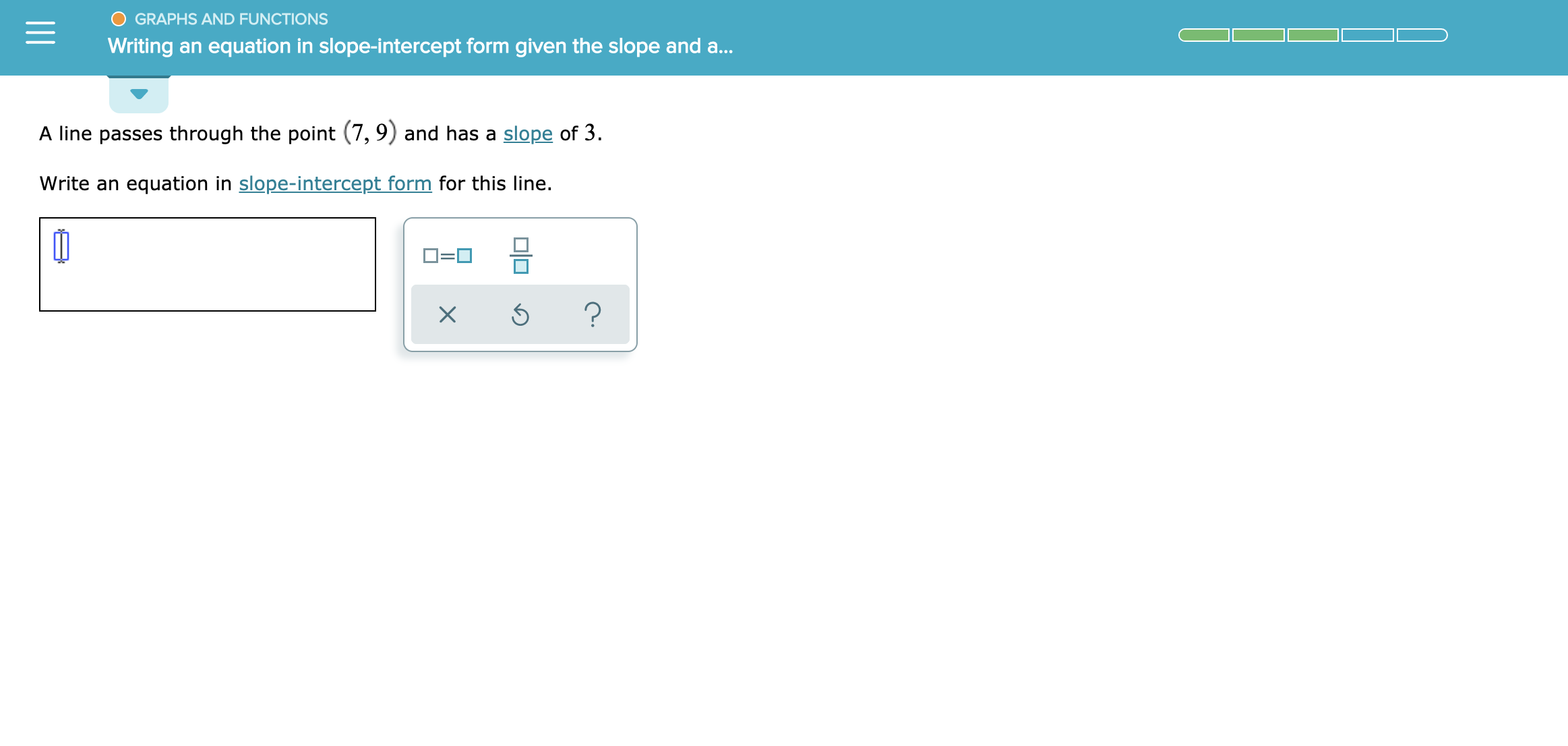# GRAPHS AND FUNCTIONSWriting an equation in slope-intercept form given the slope and a...A line passes through the point (7,9) and has a slope of 3Write an equation in slope-intercept form for this line.?

Question
3 views

See attachmenthelp_outlineImage TranscriptioncloseGRAPHS AND FUNCTIONS Writing an equation in slope-intercept form given the slope and a... A line passes through the point (7,9) and has a slope of 3 Write an equation in slope-intercept form for this line. ? fullscreen
check_circle

Step 1

Given information:

A line passes through the point (7,9) and has a slope of 3.

Step 2

Calculation:

The equation of line in slope intercept form is shown below:

Step 3

Here, x1=7 , y1=9 and m=3

Subsitute the value of x1, y1 a...

### Want to see the full answer?

See Solution

#### Want to see this answer and more?

Solutions are written by subject experts who are available 24/7. Questions are typically answered within 1 hour.*

See Solution
*Response times may vary by subject and question.
Tagged in

### Other